# TROON ESTATES 1050 WATERLOO STREET

### \$369,900

••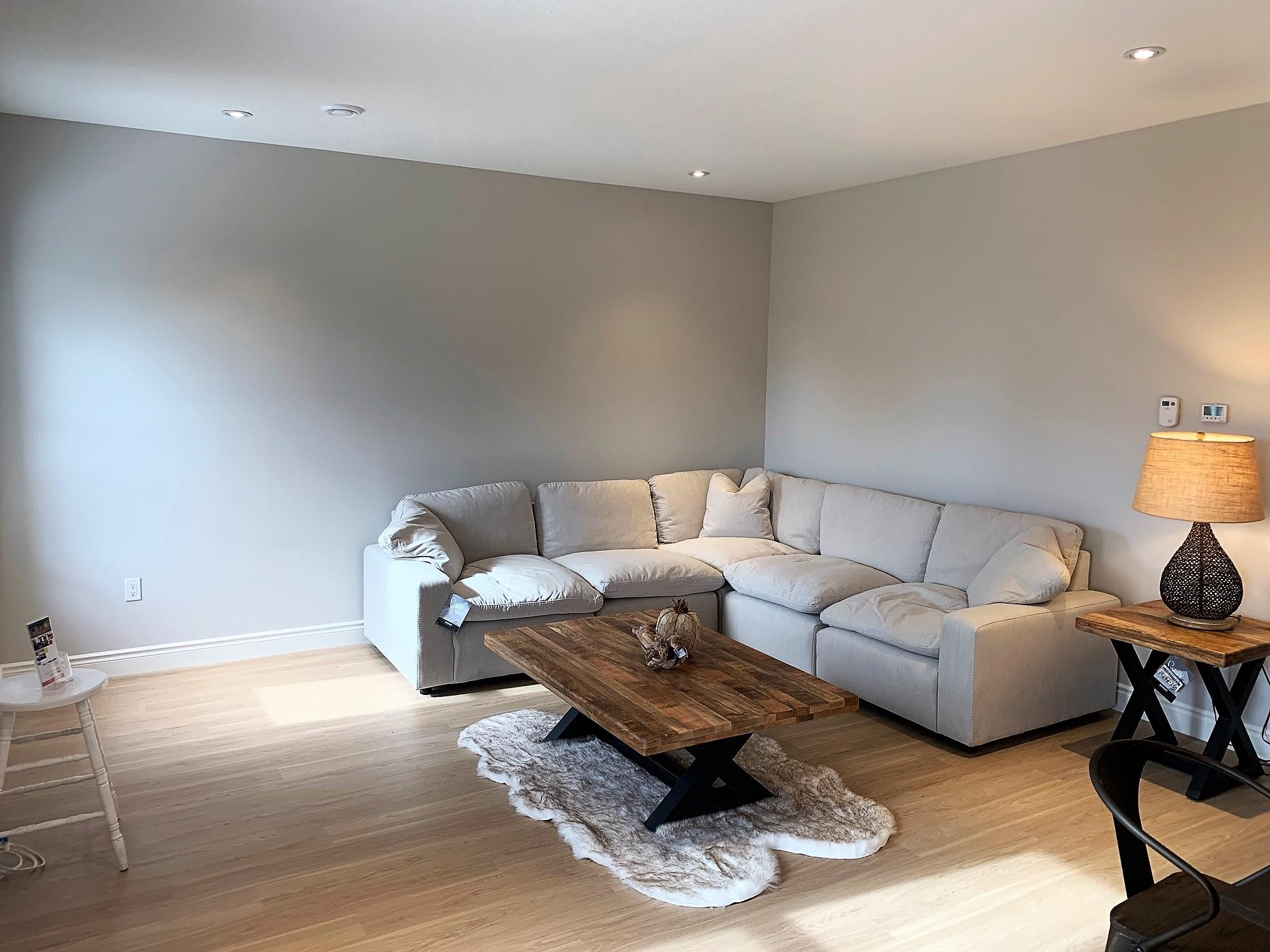•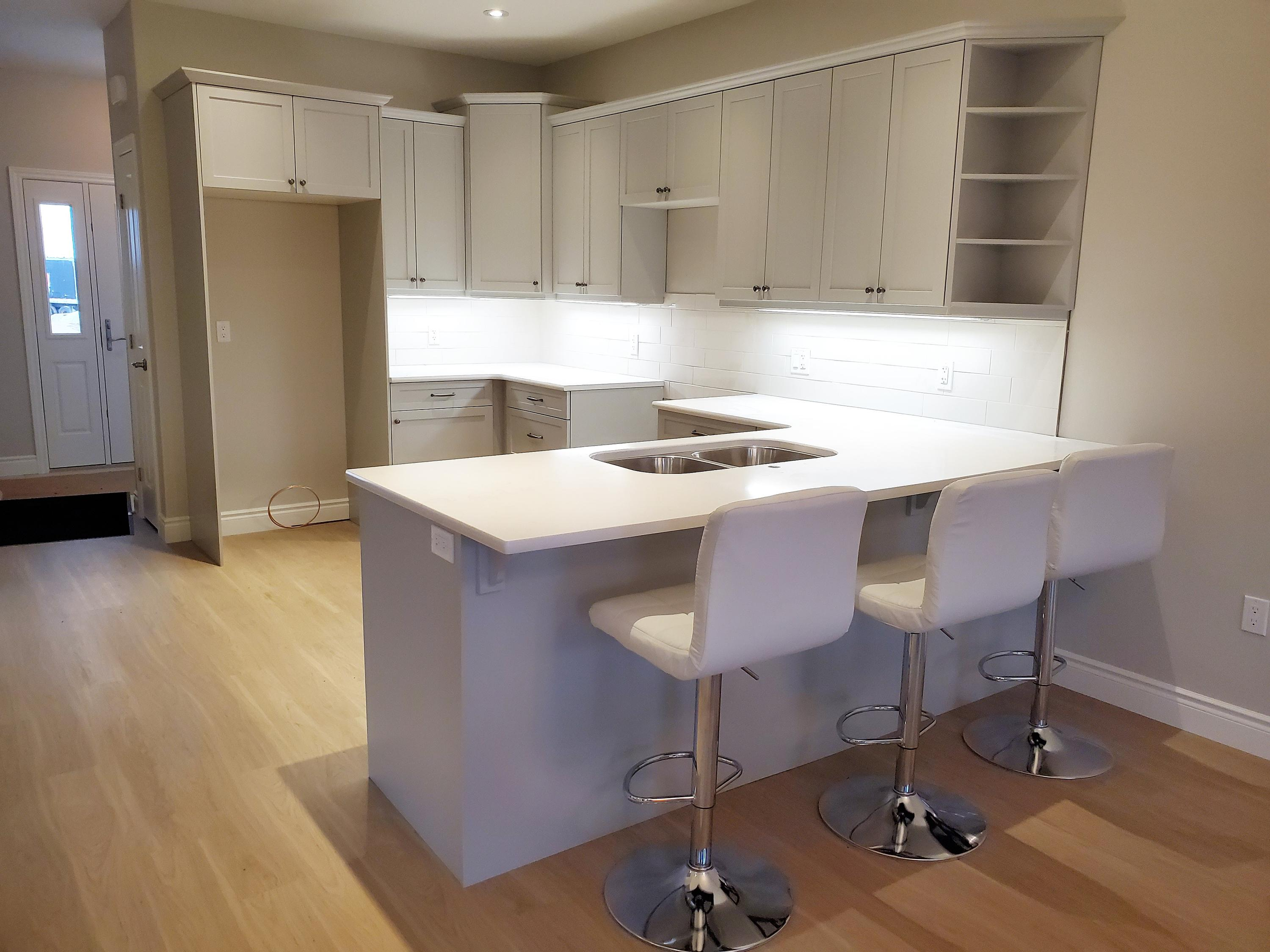••••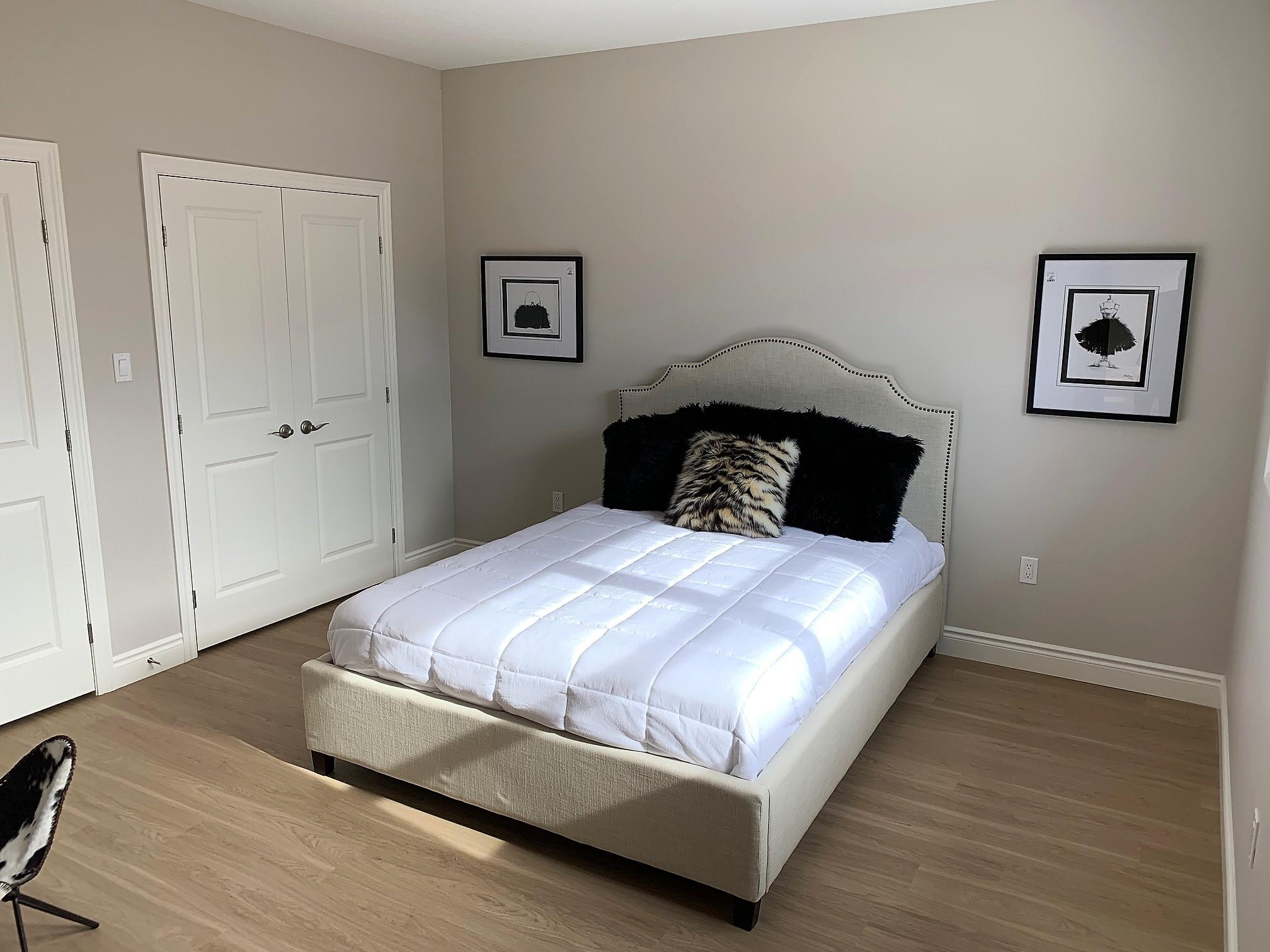•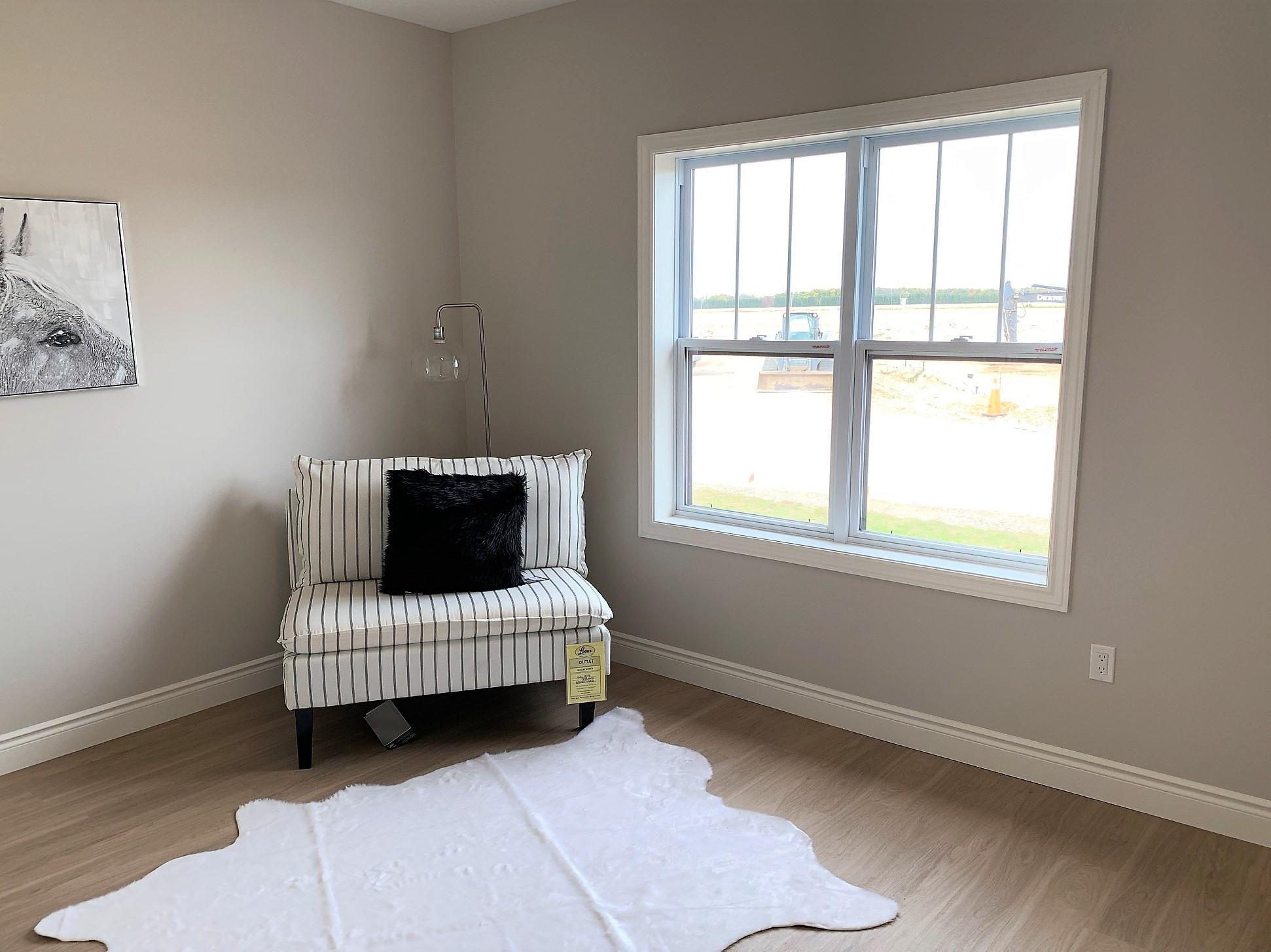•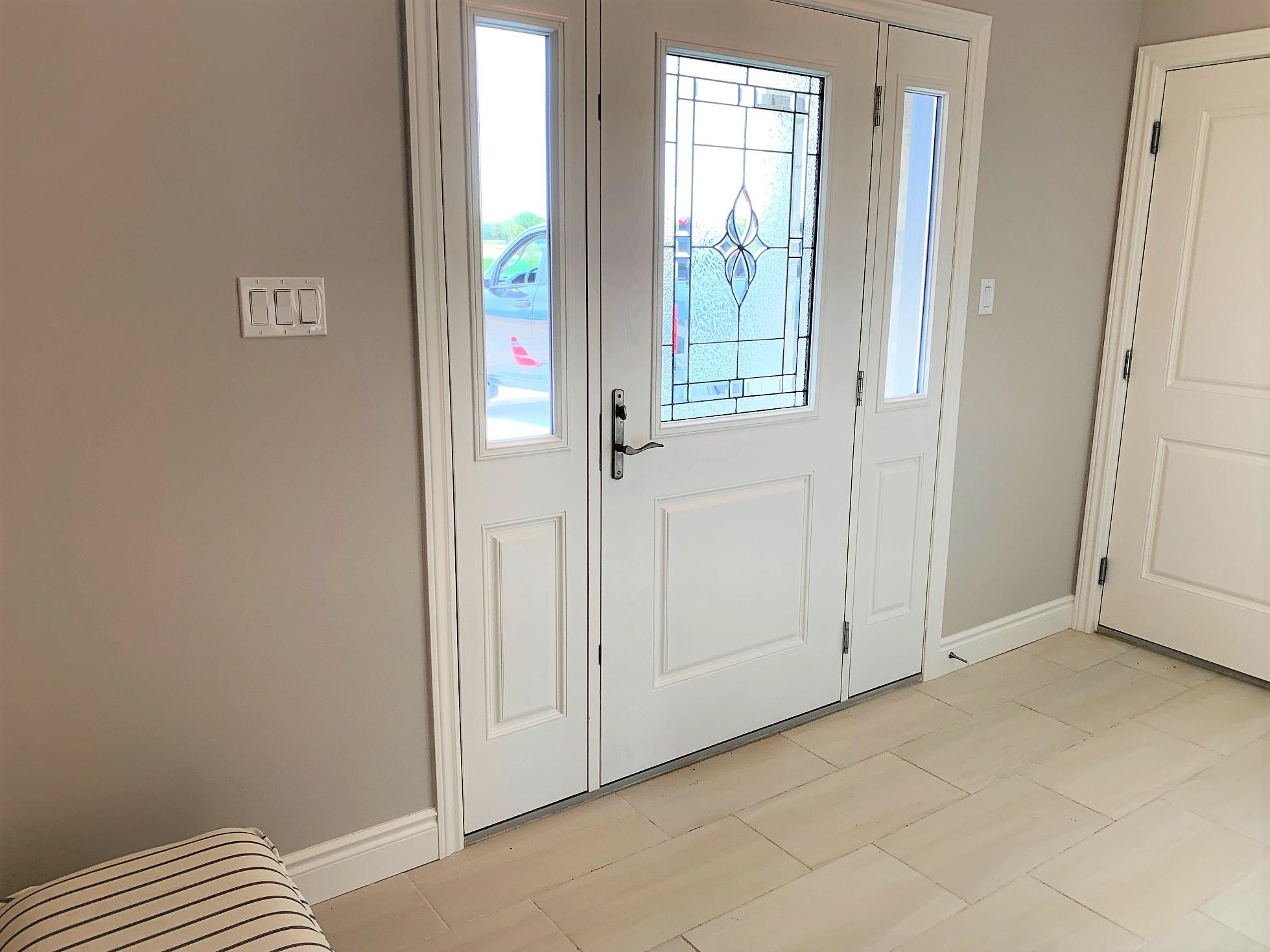•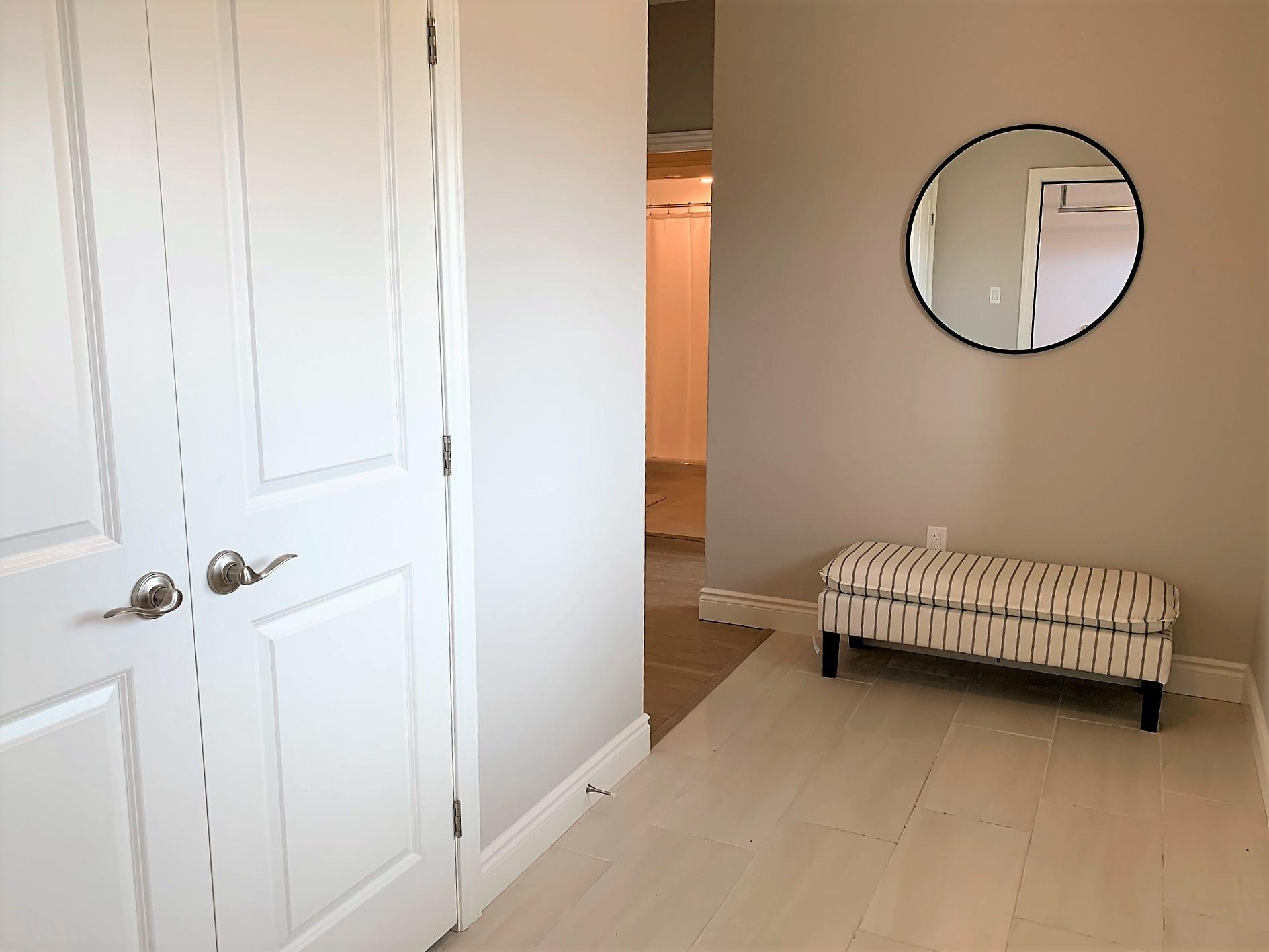••Model Unit (Model 3)
Model Unit (Unit 3)
Model Unit (Unit 3)
Model Unit (Unit 3)
Model Unit (Unit 3)
Model Unit (Unit 3)
Model Unit (Unit 3)
Model Unit (Unit 3)
Model Unit (Model 3)Model Unit (Model 3)Model Unit (Unit 3)Model Unit (Unit 3)Model Unit (Unit 3)Model Unit (Unit 3)Model Unit (Unit 3)Model Unit (Unit 3)Model Unit (Unit 3)Model Unit (Model 3)